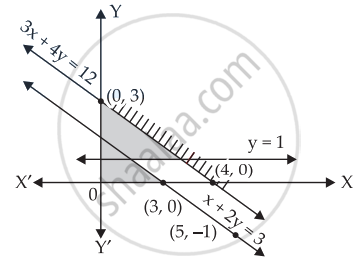# Show that the following system of linear inequalities has no solution x + 2y ≤ 3, 3x + 4y ≥ 12, x ≥ 0, y ≥ 1 - Mathematics

Chart
Graph
Sum

Show that the following system of linear inequalities has no solution x + 2y ≤ 3, 3x + 4y ≥ 12, x ≥ 0, y ≥ 1

#### Solution

Given that: x + 2y ≤ 3, 3x + 4y ≥ 12, x ≥ 0, y ≥ 1

Let x + 2y = 3

⇒ x = 3 – 2y

 x 3 1 5 y 0 1 –1

Now putting (0, 0) in x + 2y ≤ 3

0 + 0 ≤ 3

0 ≤ 3 True

Therefore, the shading will be towards (0, 0)

Consider the in equation 3x + 4y ≥ 12

Let 3x + 4y = 12

 x 0 4 2 y 3 0 1.5

Putting (0, 0) in 3x + 4y ≥ 12

0 + 0 ≥ 12

0 ≥ 12 False

Therefore, shading will be on the opposite side of the graph of line.

x ≥ 0 ⇒ Positive side of y-axis will be shaded.

And y ≥ 1 ⇒ Upperside of y = 1 will be shaded.

Let us now draw the graph.

It is clear from the graph that there is no common shaded region.

Hence, the solution is null set.Concept: Solution of System of Linear Inequalities in Two Variables
Is there an error in this question or solution?

#### APPEARS IN

NCERT Mathematics Exemplar Class 11
Chapter 6 Linear Inequalities
Exercise | Q 16 | Page 108

Share